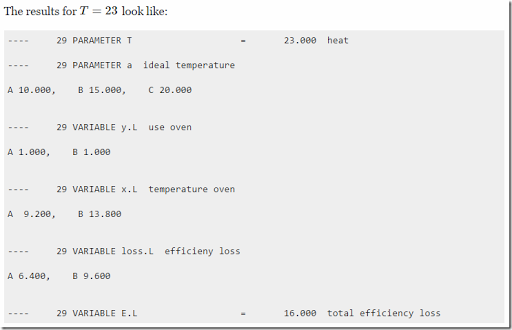## Tuesday, February 16, 2016

### Two nonlinear formulations

##### Problem Description

I am not at all sure the physics make sense, but let’s focus on the mathematics of this model.

##### Formulation A

An MINLP formulation could be:The idea is:

• If $$y_i=0$$ (oven is turned off) we force $$x_i=0$$ (equation Off). Also we have $$loss_i=0$$ as we multiplied the loss function by $$y_i$$.
• If $$y_i=1$$ (oven is turned on), we let the solver decide on $$x_i$$. In almost all cases it will choose a value > 0 as it wants to be somewhat close to $$a_i$$. But if $$x_i$$ would become zero something special happens: suddenly $$y_i=0$$ becomes a better solution.
• We don’t have to model explicitly $$x_i=0 \implies y_i=0$$. If $$x_i=0$$ then the objective would have a preference for $$y_i=0$$ as this will improve the objective.

This model is no longer quadratic. For small instances like this we can use a global solver without a problem.##### Formulation B

If we can make the problem quadratic and convex we can solve the problem with many more solvers. So here is a formulation that does just that:Here we have:

• If $$y_i=0$$ (oven is turned off) we force $$x_i=0$$ (equation Off).  The slack $$s_i$$ is left floating. This will cause the algorithm to choose a slack such that the loss is zero.
• If $$y_i=1$$ (oven is turned on) we force $$s_i=0$$ (equations On1,On2).

As this model is convex all MIQP solvers can handle this.

##### Conic formulation

For a compact conic formulations of this problem see: http://blog.mosek.com/2016/03/reformulating-non-convex-minlp-as-misocp.html